# Logistic Growth Model of the COVID-19 Pandemic to Decide When to Start the Lockdown

Logistic Growth Model of the COVID-19 Pandemic to Decide When to Start the Lockdown
LRIT Faculty of Sciences Mohamed 5 University, Rabat BP 1014 RP, Morocco

LTI Laboratory, ENSAJ Higher School of Technology of Sidi Bennour, Chouaib Doukkali University, El Jadida BP 1116, Morocco

Corresponding Author Email:
salwalagdali@gmail.com
Page:
26-30
|
DOI:
https://doi.org/10.18280/rces.070202
21 April 2020
|
Accepted:
10 June 2020
|
Published:
30 June 2020
| Citation

OPEN ACCESS

Abstract:

The fast spreading of the COVID19 disease is overwhelming the hospitals, especially the intensive care units. And this is causing a scary crisis in the health care systems and is rising the fatality rate. The pandemic is increasing exponentially making more critical situations. To overcome the spread of the disease, the concerned countries are taking different measures even lockdowns. Unfortunately, even that the social distancing measures have showed a good effectiveness in reducing the transmission of the virus, countries such as Italy and Spain were kept struggling for weeks. Our aim in this paper, is to study the exponential progress of the outbreak, then fit the data to the logistic growth model which allows us to find the right time, with acceptable delay, the concerned country should take the lockdown decision. In this paper, we also discuss when countries such us Italy, Spain and France should have started the social distancing. The result show that when the number of confirmed cases reaches a threshold, the lockdown must have started 14 days earlier. The threshold is defined to not overwhelm the health care system.

Keywords:

logistic growth model, COVID-19, lockdown decision, fitting, growth rate

1. Introduction

In 8 December 2019, a novel coronavirus (Covid19) has been emerged in Wuhan, the capital of China's Hubei province. Thereafter, it spreads globally after two months of outbreak in China. According to a clinical study of infected cases, the virus causes :

• in 80 of cases mild symptoms like flu. In this instance, the patient can recover from home.
• in 13.8 severe symptoms defined as shortness of breath. If so, the patient needs hospitalization.
• in 6,1 critical symptoms defined as respiratory failure. This requires intensive care.

The low rate of critical symptoms has caused the underestimate of the virus severity. This latter can be resumed in these points:

1. 12,1% of cases are with mild or no symptoms which make their identification a difficult task.
2. The basic reproductive number of Covid19 is 2.28  which makes the number of critical cases more important.
3. Critical beds are limited which increases the mortality rate.

In this paper, we fit Italy, Spain and France data to the exponential growth model to study the spread of the virus in the three countries and the effect of the lockdown in reducing the growth rate. Then, we describe a logistic growth model to decide when start the social distancing (accordingly reducing the number of cases) in a way to not exceed the health care capacity of the concerned country.

The remainder of this article is organized as follows: Section 2, presents the data and the assumptions used in this research. Section 3, studies the exponential progress of the daily active cases in the three countries. The fitted data to the logistic growth model, is presented in section 4. Finally, we conclude our work in section 5.

2. Methods

2.1   Data

In this modelling study, we focus on the daily total coronavirus cases in Italy, Spain and France. The data were collected from worldometers web site https://www.worldometers.info/coronavirus. We study the data from Feb 15 to April 1. In 47 days, 110574 cases were confirmed in Italy, 104118 in Spain and 56989 in France.

2.2   Assumptions

In one hand, based on the collected data we assume that the daily total cases follow an exponential distribution with a positive growth rate. This assumes that as the number of cases increases the growth rate will increase too. Consequently, the virus will invade the community if no measures was taken. In the other hand, we assume that approximately 20% of cases needs intensive care. Unfortunately, the number of critical beds is limited and does not meet this need. To avoid the pressure on the countries health care system, we describe a model that study the increase of cases with taking in account the limited number of critical beds. Therefore, we assume that the total daily cases should follow a logistic growth model with N = $\frac{B}{0.2}$ where N is the capacity of the model (the number of cases that shouldn't exceeds) and B is the number of the critical beds. Which means, when the number of the cases is small, the logistic growth model behaves as an exponential model. However, when the number of cases approach to N the growth rate approach to zero. This will give us an idea on when countries should start social distancing measures to reduce the spreading of the pandemic.

3. Exponential Model

In this section, we describe the progress of the daily total confirmed cases using exponential model. This helped to characterize the early pandemic growth rate . For that, we fit the observed daily data to the exponential distribution as:

$Y(t)=Y_{0} \cdot e^{r t}$  (1)

where, Y(t) is the observed data (number of cases) at day, t=0,  Y0 is the expected initial number of cases (at t=0) and r is the growth rate of the model. We estimate the unknown parameters (Y0 and r) with the function fitnlm from the Statistical and machine learning toolbox in Matlab (https://www.mathworks.com/).

We use the above model to study the progress of the pandemic in Italy, Spain and France. The estimation of the growth rate will give us an idea about the transmission dynamics of COVID19 and so make future projections.

## 1.png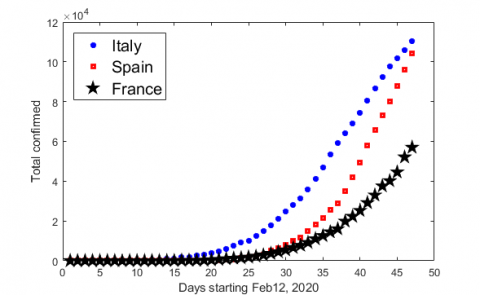Figure 1. The progress of the COVID19 daily cases

3.1 Italy

Italy has the worst scenario of the pandemic progress in Europe. As shown in Figure 1, in 47 days (from Feb 15 to Apr 1) it reached 110574 infected cases starting with 3 cases. It means that as the number of infected cases increases as the rate of pandemic transmission increases. Therefore, the cumulative cases number will increase exponentially in time.

Figure 2 shows the observed cumulative cases fitted to the exponential model Eq. (1). As we can see, the model fits the data well. Therefore, it allows us to study the different phases of infection. We divide the progress of infection into two phases:

• Phase 1: consists the period since the first case was emerged. It means from Feb 15 to 23 March (14 days after the lockdown).
•  Phase 2: starts from the day after 14 days from the lockdown. Which means from Mar 24 to Apr 1.

The study of these two phases reveals that the growth rate of cases per day floats between 15% to 39% and phase 2 indicates a decrease of the growth rate to 6%.

Based on the different growth rate values we can conclude that the social distancing is the key solution to mitigate the growth rate of the cumulative cases per day.

## 2.png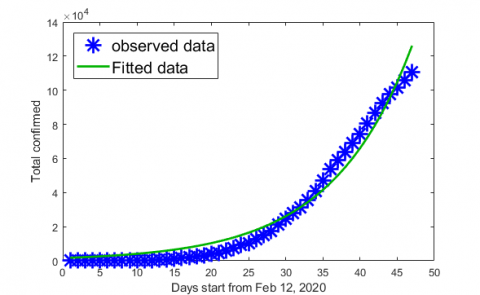Figure 2. The fitted of total confirmed in Italy to exponential model

3.2 Spain

The situation in Spain is quite similar to the Italy scenario. At Feb 15, Spain reported 2 cases, as of Apr 01, there had been 104118. The fitted collected data to the given exponential model is shown in Figure 3.

## 3.png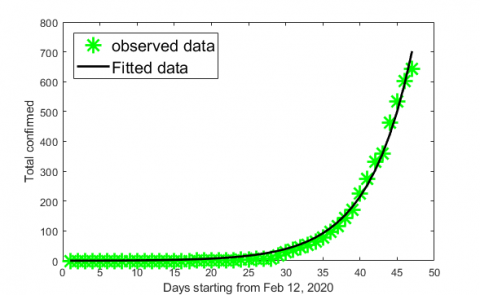Figure 3. The fitted of total confirmed in Spain to exponential model

The model fits the data well with an average growth rate of 20% cases per day. It means, the cumulative number of cases will double every 4 days.

On Mar 15, Spain has declared an absolute quarantine. We study the effect of this decision 14 days after, which means from Mar 31. The estimation of the growth rate in the period between Mar 31 and Apr 09 gives a value around 5%. Consequently, the number of cases will double every 20 days. So, based on this study, Spain will reach 191846 cases at Apr 21 instead of Apr 05.

3.3 France

Compared to Italy and Spain, France has a better scenario but it doesn't mean it is in a good situation. As shown in Figure 1, after 47 days France has reported 56989 confirmed cases, which means that the speed of the pandemic transmission is clearly an exponential growth. Our model fits the collected data from Feb 15 when it was just 12 confirmed cases Figure 4.

## 4.png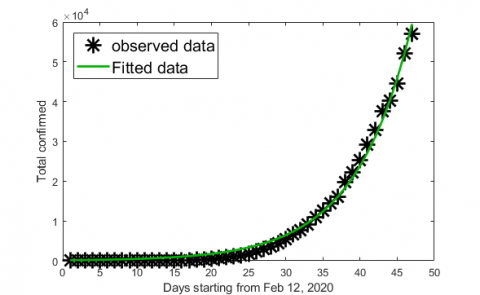Figure 4. The fitted of total confirmed in France to exponential model

The average growth rate of the model is 16%. Which means that the total confirmed cases double every 6 days. Hence, the situation will be catastrophic as the number of confirmed cases increases. In the aim of avoiding such situation, the government of France declared an absolute quarantine on Mar 17. This will help to reduce the dynamic of the virus as proved by the model. The growth rate after 14 days of lockdown has mitigated to 9%. Therefore, it just means that probably the confirmed cases will double every 11 days.

4. Logistic Growth Model

In this section, our aim is to describe a model that allows us to find the right time countries should start a lockdown. Thus, the number of final cases does not exceed the health care capacity of countries. Therefore, we need a model that acts like an exponential one at first, but it has a reduced growth rate when the number of cases approaches to the health care capacity. Hence, we fit our data to a logistic growth model  described by the Eq. (2):

$Y(t)=\frac{N}{1+A e^{-R x}}$  (2)

where, Y(t) is the number of confirmed cases at day t, N is the maximum number of cases for which the health care capacity is not exceeded, A is the initial number of cases and R is the growth rate. We use the above model to find the optimal number of cases for which the countries should start a lockdown and so the number of final cases does not exceed the health care capacity of the three countries. Notably, the number of critical beds in Italy, France and Spain is respectively 7750, 7540 and 4479 . Therefore, the maximum infected cases that are manageable for these three countries is respectively 38700, 37700 and 22395.

4.1 Italy

In less than three weeks, the coronavirus has overloaded the health care system all over northern Italy. Even hospitals in developed countries with the world's best health care risk becoming triage wards, forcing ordinary doctors and nurses to make extraordinary decisions about who may live and who may die . To reduce the virus transmission, Italy has declared a total quarantine on 9 March., Although the average growth rate has decreased to 6% (paragraph 2.3.1.), the future situation is still critical as the total number of confirmed cases is too high. That's because the growth rate is proportional to the number of cases. To avoid such situation in the second wave of infection, we fit Italy's data to the above logistic growth model where the number of maximum cases (38770 cases) is the capacity of the model.

## 5.png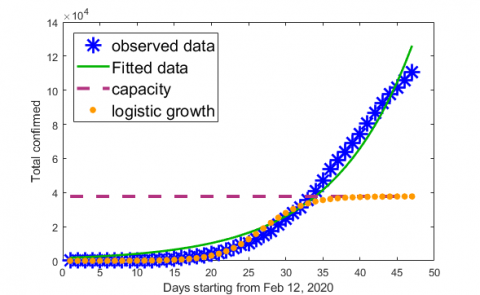Figure 5. Fitted Italy's data to the logistic growth model

As shown in Figure 5, at the early stage, the fitted data increases rapidly until the number of cases reaches$\frac{38700}{2}$=19385 for which the growth rate is at its maximum. Then, the growth rate remarkably slows. As detailed in Figure 6.

## 6.pngFigure 6. The daily growth rate of Italy's total confirmed cases

When the number of the cases reached 19385, the growth rate declines from 0.15% to 0.11%. Consequently, to not overload the health care system, the growth rate should decrease before the number of cases reaches 19385 cases. Hence, if we assume that on average the number of cases doubles every 5 days, this is mean that strict social distancing should be started 15 days before 19385 cases is reached. In other words, when 2423 cases is reached. Of course, the tests have to be done as soon as possible on a large population.

4.2 Spain

Spain, is the second-most affected country in Europe after Italy. In the aim to slow the transmission of the disease, Spain has declared a lockdown at 14 March. Although the average growth rate has decreased to 5% the country is still struggling to slow the spread of the virus due to the high total confirmed cases even after the lockdown was launched. Hence, it is important to have an idea about the time to start social distancing. For this reason, we fit Spain's data to the logistic growth model where the number of maximum cases (22395 cases) is the capacity of the model.

## 7.png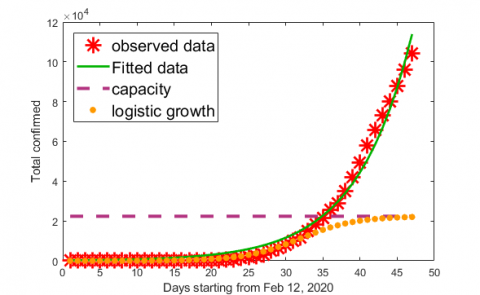Figure 7. Fitted Spain's data to the logistic growth model

As shown in Figure 7, the growth rate remarkably slows when the number of cases reached $\frac{22395}{2}=111997$.

## 8.png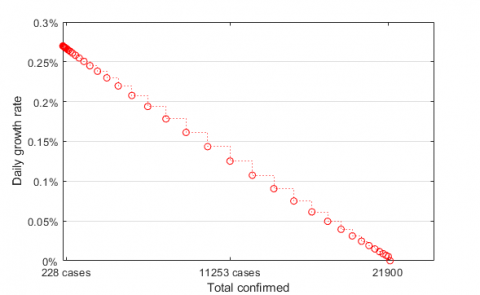Figure 8. The daily growth rate of Spain's total confirmed cases

As we can observe in Figure 8, when the number of the cases reaches 11197, the growth rate declines from 0.13% to 0.1%. Consequently, to not exceed the health care capacity, the growth rate should decrease to 0.1 as the number of cases reach 11197 cases. Hence, if on average the number of cases doubles every 5 days, this means that strict social distancing should have been started 15 days before 11197 cases can be reached. In other words, when 1400 cases are reached.

4.3 France

In the aim to reduce the spread of the virus, the government of France declared an absolute quarantine on Mar 17. Which helped to reduce the growth rate to 9% but not to achieve yet a lower number of active cases. The fit of France's data to the logistic growth model where the number of maximum cases (37700 cases) is the capacity of the model will help us to know the correct time to start the social distancing.

As shown in Figure 9, the model increases rapidly at first but when it reaches $\frac{37700}{2}=18850$ cases the progress of the graph starts to slow remarkably. which means, the growth rate starts to slow at this point (from 0.11% to 0.08%) as observed in Figure 10.

## 9.png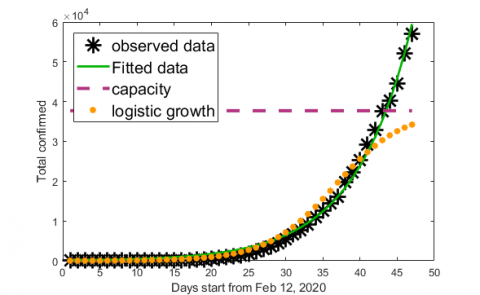Figure 9. Fitted France's data to the logistic growth model

## 10.png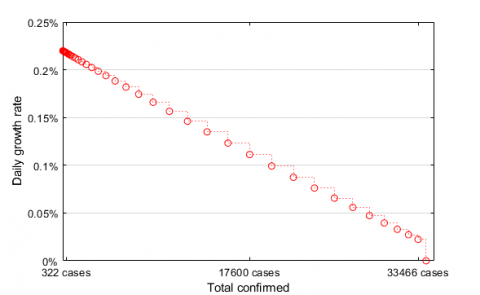Figure 10. The daily growth rate of France's total confirmed cases

Based on our results, we conclude that the strict social distancing should be started 15 days before reaching 18850 cases. Which means, when 2356 cases are reached.

5. Conclusions

In this paper, we have studied the exponential progress of the total daily cases in Italy, Spain and France. The study has showed that if no measures are token, the hospitals will be overwhelmed. For this reason, the countries has declared a lockdown to reduce the spread of the virus. Unfortunately, even the transmission of the virus has been reduced, the countries kept struggling for weeks because of the delay in the lockdown decision. This is why, in this research we have described a logistic growth model to decide the moment when the countries should start the social distancing measures with an accepted delay. The result show that when the number of confirmed cases reaches a threshold, the lockdown must have started 14 days earlier. The threshold is defined to not overwhelm the health care system and is calculated by the fraction of the number of the total cases that the model should not exceed by 2. For example, Italy should have started the lockdown 14 days before reaching 2423 cases.

References

 World Health Organization. (2020) Report of the WHO-China Joint Mission on Coronavirus Disease 2019 (COVID-19).

 Zhang, S., Diao, M., Yu, W., Pei, L., Lin, Z., Chen, D. (2020). Estimation of the reproductive number of Novel Coronavirus (COVID-19) and the probable outbreak size on the Diamond Princess cruise ship: A data-driven analysis. International Journal of Infectious Diseases, 201-204. https://doi.org/10.1016/j.ijid.2020.02.033

 Viboud, C., Simonsen, L., Chowell, G.A. (2016). generalized growth model to characterize the early ascending phase of infectious disease outbreaks. Epidemics Journal, 15: 27-37. https://doi.org/10.1016/j.epidem.2016.01.002

 Haberman, R. (1998) Mathematical models: mechanical vibrations, population dynamics, and traffic flow. SIAM. Vol. 21.

 Rhodes, A., Ferdinande, P., Flaatten, H., Guidet, B., Metnitz, P.G., Moreno, R.P. (2012). The variability of critical care bed numbers in Europe. Intensive Care Medicine, 38(10): 1647-1653. https://doi.org/10.1007/s00134-012-2627-8

 Horowitz, J. (2020). Italy’s health care system groans under coronavirus--a warning to the world. The New York Times. Vol. 12.